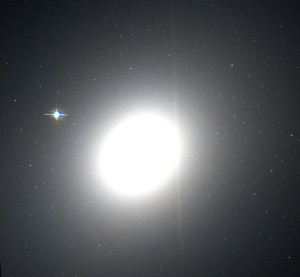# Approximating galaxies as spheres

A couple days ago I compared different ways of approximating Earth and other ellipsoids by spheres. The earth is so nearly spherical that the difference in the approximations would only matter when you need fairly high accuracy. Elliptical galaxies, however, can be much more eccentric than Earth and so the difference in approximation approaches can matter more.

The Hubble classification of elliptical galaxies uses a scale E0 through E7 where the number following ‘E’ is 10(1 – b/a) where a is the major semi-axis and b is the minor semi-axis. An E0 galaxy is essentially spherical. The most common classification is near E3. The limit is believed to be around E7.The image above is a photo of Messier 49, an E4 galaxy, taken by the Hubble telescope.

For an E3 galaxy, the minor and major axes are around 7 and 10 in some unit. The average of these is 8.5. A sphere with the same volume would have radius 8.88 and a sphere with the same surface area would have radius 8.98, about 5.7% larger than the average of the axes.

For an E7 galaxy, the minor and major axes would have a ratio of 3 to 10. This gives an average of 6.5. Matching volumes gives a radius of 6.69 and matching surface area gives a ratio of 7.67, about 18% larger than the average of the axes.

## 2 thoughts on “Approximating galaxies as spheres”

1. Ewan Cameron

Not quite sure how I stumbled over to this part of the web, but you might be interested in this paper below, describing a useful mathematical “trick” for studying elliptical galaxies which fell as manna from heaven out of Mathematica one day :-) :
http://arxiv.org/abs/1009.4713